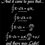# Harmonics

$$\sin(x)$$ and $$\cos(x)$$ are harmonic functions.Their series can be given as,

\begin{aligned} \sin x &=& x - \dfrac{x^3}{3!} + \dfrac{x^5}{5!} - \dfrac{x^7}{7!} + \cdots = \sum_{n=0}^\infty \dfrac{(-1)^n x^{2n+1}}{(2n+1)!} \\ \cos x &=& 1 - \dfrac{x^2}{2!} + \dfrac{x^4}{4!} - \dfrac{x^6}{6!} + \cdots = \sum_{n=0}^\infty \dfrac{(-1)^n x^{2n}}{(2n)!} \end{aligned}

Here, we can see that both the above series have maximum magnitude -: $1$. The speciality of both the functions is that there is $+$ and $-$ signs alternatively. Thats why for any big or small values of $x$, both the above series converges to $1$ in magnitude.

Now, if we look at the expansion of $e^{(-x)}$,

$e^{(-x)} = 1-x+\dfrac{x^2}{2!}-\dfrac{x^3}{3!}+\dfrac{x^4}{4!}-\dfrac{x^5}{5!}+...$

Here also the same situation occurs. But why it is not a function like $sine$ and $cos$? Why its value is not repeated like $sine$ and $cos$?Note by Akash Shukla
4 years, 3 months ago

This discussion board is a place to discuss our Daily Challenges and the math and science related to those challenges. Explanations are more than just a solution — they should explain the steps and thinking strategies that you used to obtain the solution. Comments should further the discussion of math and science.

When posting on Brilliant:

• Use the emojis to react to an explanation, whether you're congratulating a job well done , or just really confused .
• Ask specific questions about the challenge or the steps in somebody's explanation. Well-posed questions can add a lot to the discussion, but posting "I don't understand!" doesn't help anyone.
• Try to contribute something new to the discussion, whether it is an extension, generalization or other idea related to the challenge.

MarkdownAppears as
*italics* or _italics_ italics
**bold** or __bold__ bold
- bulleted- list
• bulleted
• list
1. numbered2. list
1. numbered
2. list
Note: you must add a full line of space before and after lists for them to show up correctly
paragraph 1paragraph 2

paragraph 1

paragraph 2

[example link](https://brilliant.org)example link
> This is a quote
This is a quote
    # I indented these lines
# 4 spaces, and now they show
# up as a code block.

print "hello world"
# I indented these lines
# 4 spaces, and now they show
# up as a code block.

print "hello world"
MathAppears as
Remember to wrap math in $$ ... $$ or $ ... $ to ensure proper formatting.
2 \times 3 $2 \times 3$
2^{34} $2^{34}$
a_{i-1} $a_{i-1}$
\frac{2}{3} $\frac{2}{3}$
\sqrt{2} $\sqrt{2}$
\sum_{i=1}^3 $\sum_{i=1}^3$
\sin \theta $\sin \theta$
\boxed{123} $\boxed{123}$

Sort by:

The problem is that $\sin x$ and $\cos x$ do not converge, they are just bounded between $[-1,1]$.
Proving this (and periodicity) is not direct from the series expansions, irrespective of the $+$ and $-$ signs.
$e^{-x}$ is still a function, however.

- 4 years, 3 months ago

Thank you, Sir. I think the power changes the property. As in $sin$ and $cos$ the powers are odd and even, so even for any higher value of $x$, due to + and -, it will be bounded to $-1$ to $1$.

- 4 years, 3 months ago

Of course it does change the property, but how can you say that it's bounded just by looking at the signs?

- 4 years, 3 months ago

If + and - are not alternate or if + or - sign are repeated after two terms then I think it will not repeat its value.

- 4 years, 3 months ago